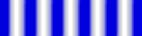# The Meaning of the Wave

At this point we have a bit of a mystery. We've seen that electrons, under some circumstances will behave like particles, and under other circumstances will behave like a wave. But a wave of what? A wave implies that something is oscillating. In a water wave, the water itself goes up and down. In a sound wave, air pressure oscillates as the wave passes through. In a light wave, there's an electric and magnetic field that oscillate, as Maxwell and Hertz showed. With an electron wave, there's got to be something oscillating, but what?

To answer this, let's go back and reconsider Young's experiment. Stripping away the details, we shine light through a couple of slits, we place a screen behind the slits to observe the result, and an interference patternappears on the screen. The screen is brightest where the amplitude of the wave striking the screen is greatest, and where the amplitude is small, the screen is dim. (Amplitude is a word physicists use to describe the size of the oscillations in a wave. For example, in a water wave, the amplitude refers to the height of the wave. In a light wave, the amplitude refers to the size of the fluctuations in the electric field, etc.)

Now let's think of this in terms of particles (photons). The photons pass through the slits and strike the screen. (Then they bounce off in all directions; some of them reach our eyes, and so we're able to see that the screen is lit up.) The bright stripes on the screen are bright because lots of photons are hitting there, while places where very few photons strike will be dim.

Finally, let's consider a single photon. It passes through the slits and strikes the screen. Where is it going to hit? Well, they're hitting all over the place, so we really can't tell for sure where this one is going to go. On the other hand, we can state with confidence that it will probably hit one of the places on the screen where it's bright, since that's where most of them are hitting. (That's why it's bright, remember?) And the brightness of the light on the screen depends on the amplitude of the light wave at that point. (To be more specific, the brightness depends on the square of the amplitude.)

So we've linked the amplitude of the wave to the number of photons in that area, and the number of photons in an area to the probability of any given photon being in that area. This is how the wave and the particle are related in waveşparticle duality. The amplitude of the wave tells us the probability of a particle being there. We can think of the wave as a wave of probability.

So Young's experiment works like this. Suppose instead of a whole beam of light, we launch just a single photon through the slits and observe where it hits the screen. From the time the photon passes through the slits, we don't know where it is until we "measure" its location by letting it hit the screen. In the meantime, the wave travels from the slits to the screen, and the amplitude of the wave determines the probability of where we'll find the photon when we do measure its location. I've put this last bit in bold print, because it's a central fact of waveşparticle duality, whether we're dealing with photons, electrons, neutrinos, or whatever.

From many waves, one
Previous page
The Page of Uncertainty